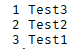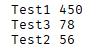# How to use table.sort?

How do you go about using table.sort() in a table like this:

`````` {
["Test1"] = 420,
["Test2"] = 1000000,
["Test3"] = 48189,
}
``````

I have tried using the following:

``````table.sort(Table, function(a, b)
return a > b
end)
``````

but it doesn’t work. I think a and b are Test1, Test2, etc but it doesn’t want to print a and b.

2 Likes

You could detect the number inside the `Test` string and use that in the sorting function,
to detect numbers - use the special key “%d”.

I am a little new to using % in scripts. How do I use this in this case?

https://www.lua.org/pil/19.3.html
The 2nd paragraph goes over that you can only use this on arrays, not dictionaries. What you are doing is using it on a dictionary.

They show an example of transfering a dictionary over to an array and then back. While using table.sort in between.

You need to realize that you’re using a dictionary, and this function works only on arrays.

Here’s an example on how to use it :

This will return you the numbers in order [larger to smaller]

``````local tab = {1,2,5,6,3}

table.sort(tab,function(a,b)
return a>b
end)

for a,b in pairs(tab) do
print(a,b)
end

``````

Here is how you can use it in your case:

In this example, it’d return you the values in the table in order, from larger to smaller [it’ll detect the numbers in your values and work with that]

``````local mytab = {"Test1","Test2","Test3"}

table.sort(mytab,function(a,b)
end)

for i,v in pairs(mytab) do
print(i,v)
end
``````4 Likes

I think you misunderstood it. What I am trying to do is sort the values that are after the Test (420, 100000 and 48189 in this case)

Well, the example you showed me above uses `dictionaries`, and that function doesnt work with that, but with `arrays`.

1 Like

But! Here is a way you could do that in your case :

[I will now send an example with this applied on]

In this example, it’d sort them accordingly from higher to lower.

``````local mytab = {
{"Test1",450};
{"Test2",56};
{"Test3",78};
}

table.sort(mytab, function(a,b)
return a > b
end)

for i,v in pairs(mytab) do
print(v, v)
end

``````13 Likes

Is there any way that you can sort Instances instead of strings with this example?

Yes, wouldn`t even need to change anything.
Your table could have any number of things you would like, the Sorting is done by just comparing the Numbers and ordering the table, nothing else matters.